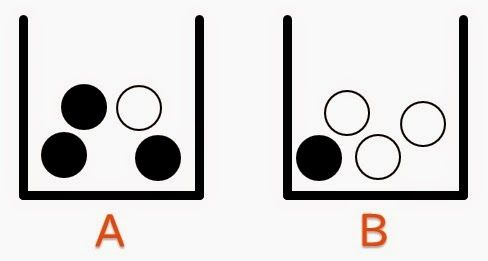Thinking in Odds

There are many ways to express something's probability. Using the usual decimal method (0 to 1) is convenient for a number of reasons, and particularly when thinking in terms of risk and expected outcomes. But it doesn't handle inference very well - inference being the process of incorporating information into one's probabilistic judgements. The reason is that the impact of a piece of information on a decimal probability differs depending on what probability you start with.Suppose you know that one of the two urns has been chosen randomly, so that the probability it's urn A is 50%. A ball is now drawn from the chosen urn - it's black - and then returned to the urn. The black ball has raised the probability that urn A was chosen from 50% to 75%. Another ball is drawn from the same urn, and is also black. This time, the black ball has only raised the probability that urn A has been chosen from 75% to 90%. The information is the same, but the effect on your probability is different. In fact the mathematical impact on probability is quite convoluted to express algebraically.

Thinking in odds makes things a bit simpler. Drawing a black ball has an evidential odds ratio of 3:1 in favour of urn A; in other words, a black ball is three times more probable if urn A has been chosen than if urn B was chosen. Starting with odds of 1:1 (i.e. 50%), the first black ball raises the odds to 3:1. The next has a similar effect, raising the odds from 3:1 (75%) to 9:1 (90%). The odds are raised by the same factor (three) in both cases, respecting the fact that the information was the same.

Expressing probabilities in terms of odds makes it easier to separate our base rate (the probability we started with) from the evidence (the new information that affects the probability). For this reason, there is growing support for the use of odds to express the evidential power of scientific experiments, as an alternative to the perpetually-counterintuitive significance test.

Of course we shouldn't forget that decimal probabilities and odds merely express the same thing in two different ways. The difference is in the way we respond to them cognitively. Broadly, it's easier to use decimal probabilities for thinking about expected outcomes and decision-making, and odds for thinking about information and inference.